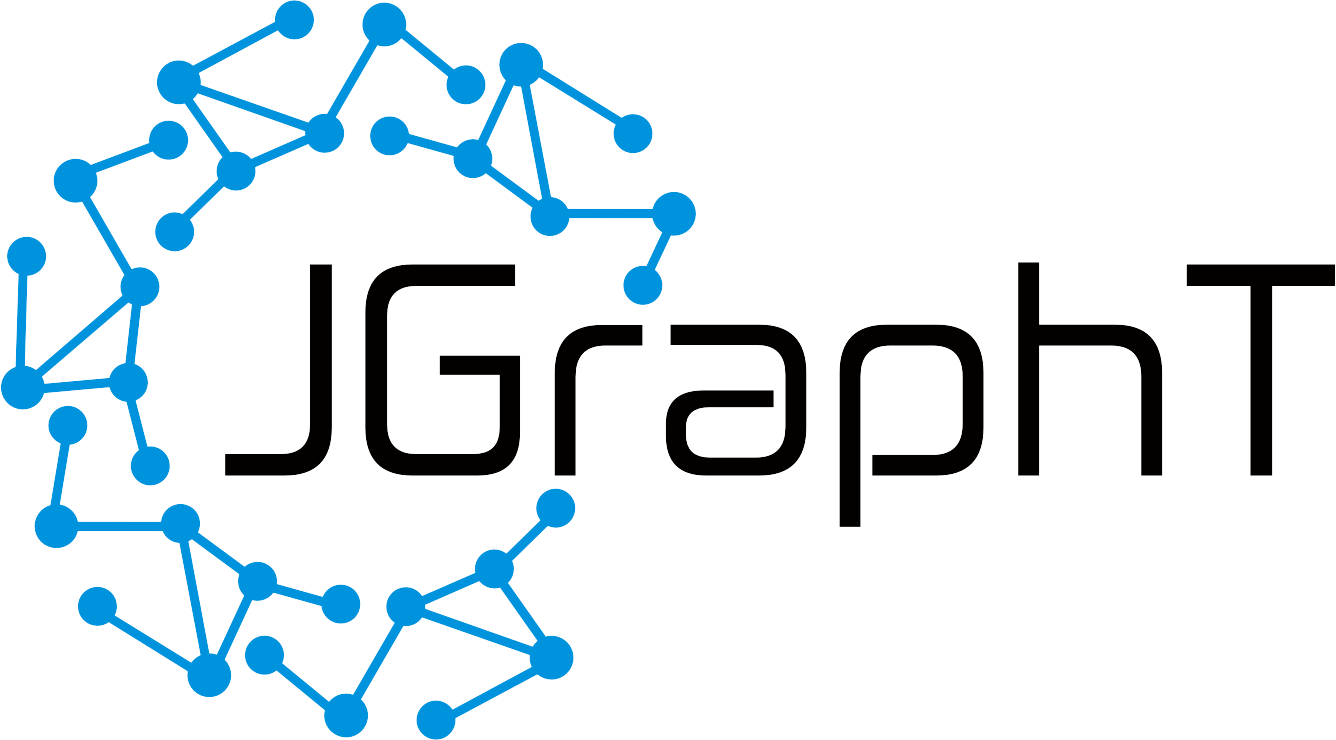< Guava Graph Adapter | JGraphTIf you are using Guava’s common.graph data structure, and would like to take advantage of an algorithm implemented by JGraphT, it’s quite straightforward to do this via the adapters supplied by JGraphT.

For example, suppose you’ve created a Guava graph as follows:

``````        MutableGraph<String> guava = GraphBuilder.undirected().build();
guava.putEdge("ul", "um");
guava.putEdge("um", "ur");
guava.putEdge("ml", "mm");
guava.putEdge("mm", "mr");
guava.putEdge("ll", "lm");
guava.putEdge("lm", "lr");
guava.putEdge("ul", "ml");
guava.putEdge("ml", "ll");
guava.putEdge("um", "mm");
guava.putEdge("mm", "lm");
guava.putEdge("ur", "mr");
guava.putEdge("mr", "lr");
``````

The graph does not have any information associated with the edges, so we can use JGraphT’s MutableGraphAdapter to view it in JGraphT:

``````        Graph<String, EndpointPair<String>> jgrapht = new MutableGraphAdapter<>(guava);
``````

Now suppose we want to find a minimum vertex cover for this graph. JGraphT supplies several algorithms for this purpose:

``````        VertexCoverAlgorithm<String> alg = new RecursiveExactVCImpl<>(jgrapht);
VertexCoverAlgorithm.VertexCover<String> cover = alg.getVertexCover();
Set<String> expectedCover = Set.of("um", "ml", "mr", "lm");
assertEquals(expectedCover, cover);
``````

Since the result is just a set of strings, it can be used to directly reference the JGraphT view as well as the underlying Guava graph.